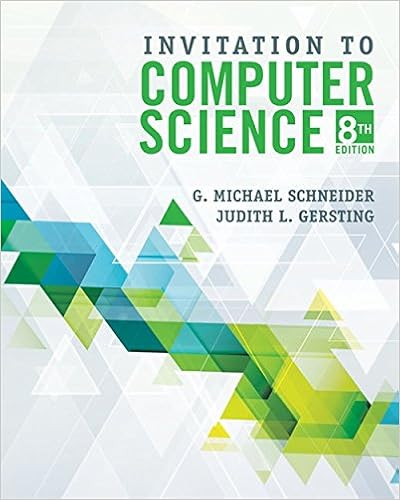# Solution a here we have and so and from theorem 4 we

• Notes
• mimi304
• 60
• 0% (2) 0 out of 2 people found this document helpful

This preview shows page 19 - 22 out of 60 pages.

##### We have textbook solutions for you!
The document you are viewing contains questions related to this textbook.The document you are viewing contains questions related to this textbook.
Chapter 3 / Exercise 1
Invitation to Computer Science
Gersting/SchneiderExpert Verified
SOLUTION (a) Here we have and So , , , , and From Theorem 4, we get (b) If we ask a computer algebra system to evaluate the sum and simplify, we obtain Now we ask the computer algebra system to evaluate the limit: We will learn a much easier method for the evaluation of integrals in the next section. Evaluate the following integrals by interpreting each in terms of areas. (a) (b) SOLUTION (a) Since , we can interpret this integral as the area under the curve from 0 to 1. But, since , we get , which shows that the graph of is the quarter-circle with radius 1 in Figure 9. Therefore (In Section 7.3 we will be able to prove that the area of a circle of radius r is .) f y s 1 x 2 y 2 1 x 2 x 2 y 2 1 f x s 1 x 2 0 y 1 0 s 1 x 2 dx y 3 0 x 1 dx v EXAMPLE 4 EXAMPLE 3 x 5 2 x 4 dx f x x 4 , a 2, b 5, x b a n 3 n x 0 2 x 1 2 3 n x 2 2 6 n x 3 2 9 n x i 2 3 i n y 5 2 x 4 dx lim n l n i 1 f x i x lim n l n i 1 f 2 3 i n 3 n lim n l 3 n n i 1 2 3 i n 4 n i 1 2 3 i n 4 2062 n 4 3045 n 3 1170 n 2 27 10 n 3 y 5 2 x 4 dx lim n l 3 n n i 1 2 3 i n 4 lim n l 3 2062 n 4 3045 n 3 1170 n 2 27 10 n 4 3 2062 10 3093 5 618.6 y 1 0 s 1 x 2 dx 1 4 1 2 4 r 2 Because is positive, the integral in Example 3 represents the area shown in Figure 8. f x x 4 FIGURE 8 x y 0 2 5 y=x\$ 300 x y 1 0 1 y= 1-≈ or ≈+¥=1 œ „„„„„ FIGURE 9
##### We have textbook solutions for you!
The document you are viewing contains questions related to this textbook.The document you are viewing contains questions related to this textbook.
Chapter 3 / Exercise 1
Invitation to Computer Science
Gersting/SchneiderExpert Verified
302 CHAPTER 4 (b) The graph of is the line with slope 1 shown in Figure 10. We compute the integral as the difference of the areas of the two triangles: The Midpoint Rule We often choose the sample point to be the right endpoint of the th subinterval because it is convenient for computing the limit. But if the purpose is to find an approximation to an integral, it is usually better to choose to be the midpoint of the interval, which we denote by . Any Riemann sum is an approximation to an integral, but if we use midpoints we get the following approximation. Midpoint Rule where and Use the Midpoint Rule with to approximate . SOLUTION The endpoints of the five subintervals are , , , , , and , so the midpoints are , , , , and . The width of the subintervals is , so the Midpoint Rule gives Since for , the integral represents an area, and the approxi- mation given by the Midpoint Rule is the sum of the areas of the rectangles shown in Figure 11. f x 1 x 0 1 x 2 0.691908 1 5 1 1.1 1 1.3 1 1.5 1 1.7 1 1.9 y 2 1 1 x dx x f 1.1 f 1.3 f 1.5 f 1.7 f 1.9 x 2 1 5 1 5 1.1 1.3 1.5 1.7 1.9 1 1.2 1.4 1.6 1.8 2.0 v EXAMPLE 5 n 5 y 2 1 1 x dx x i 1 2 x i 1 x i midpoint of x i 1 , x i x b a n y b a f x dx n i 1 f x i x x f x 1 f x n x i x i * x i * i x y 1 0 _1 3 y=x-1 (3, 2) A™ FIGURE 10 y 3 0 x 1 dx A 1 A 2 1 2 2 2 1 2 1 1 1.5 y x 1 FIGURE 11 0 x y 1 2 y= 1 x Module 4.2/7.7 shows how the Midpoint Rule estimates improve as increases. TEC n
THE DEFINITE INTEGRAL 303 At the moment we don’t know how accurate the approximation in Example 5 is, but in Section 7.7 we will learn a method for estimating the error involved in using the Midpoint Rule. At that time we will discuss other methods for approximating definite integrals.
•••